Constructing line parallel to given line

Practical Geometry
Serial order wise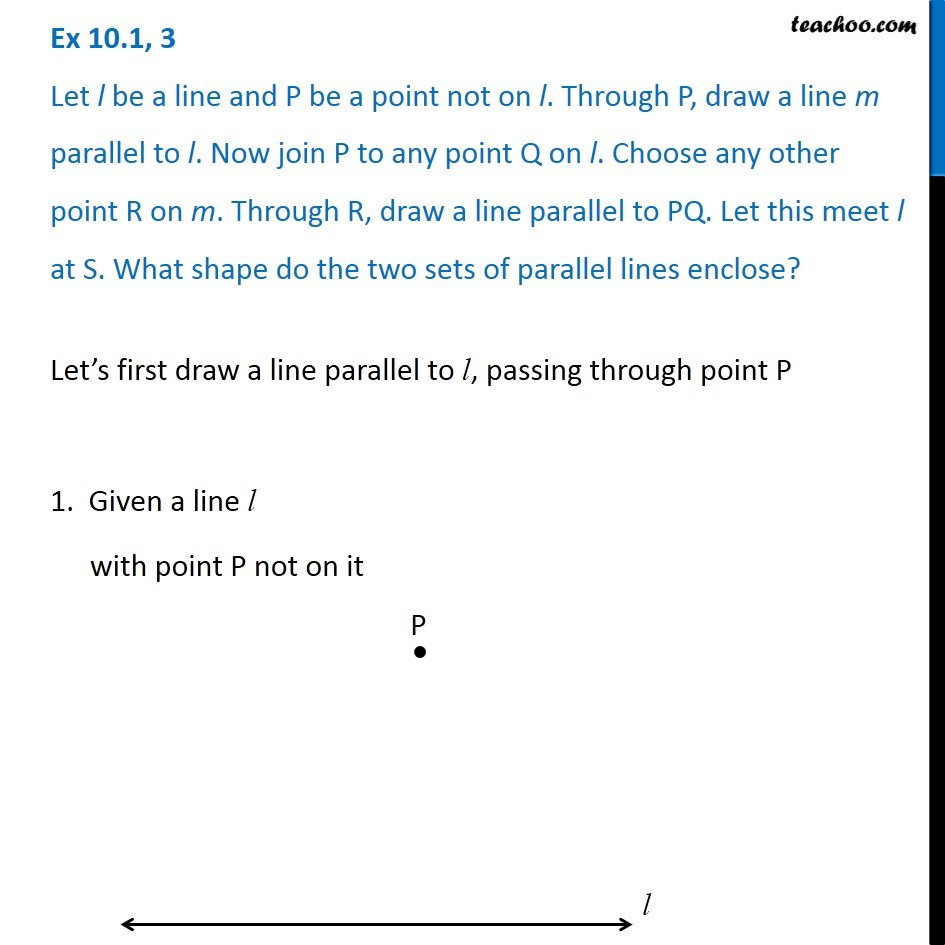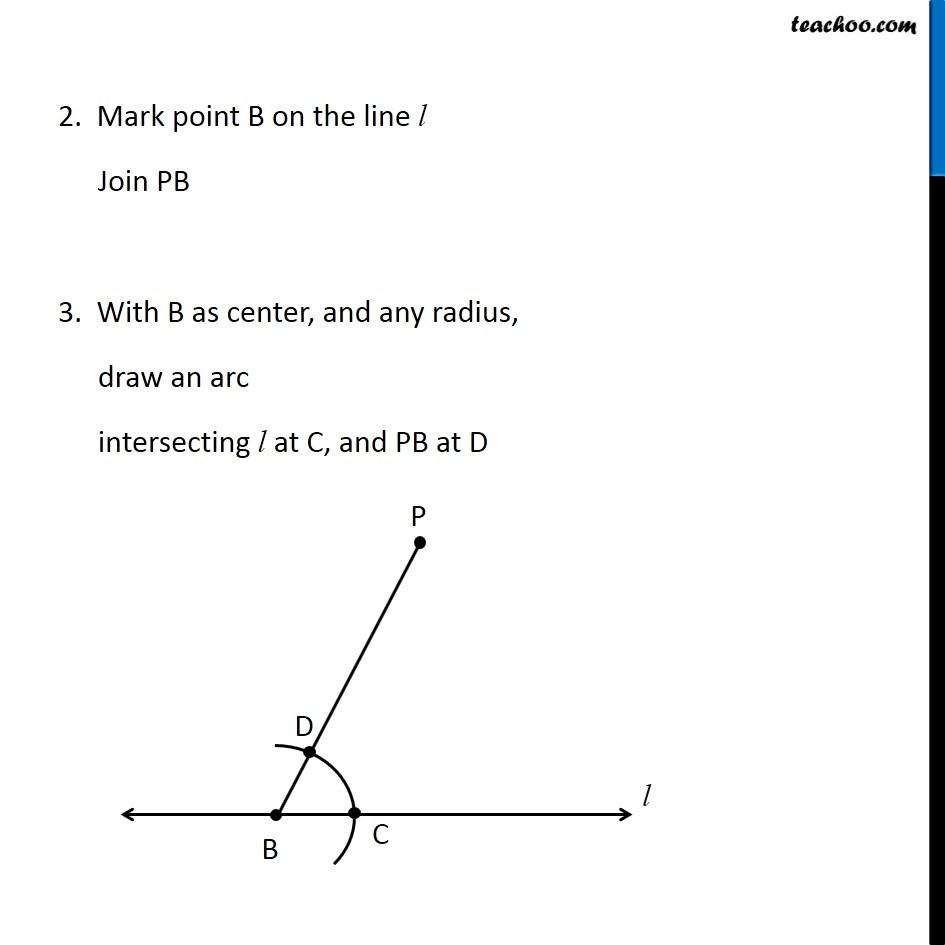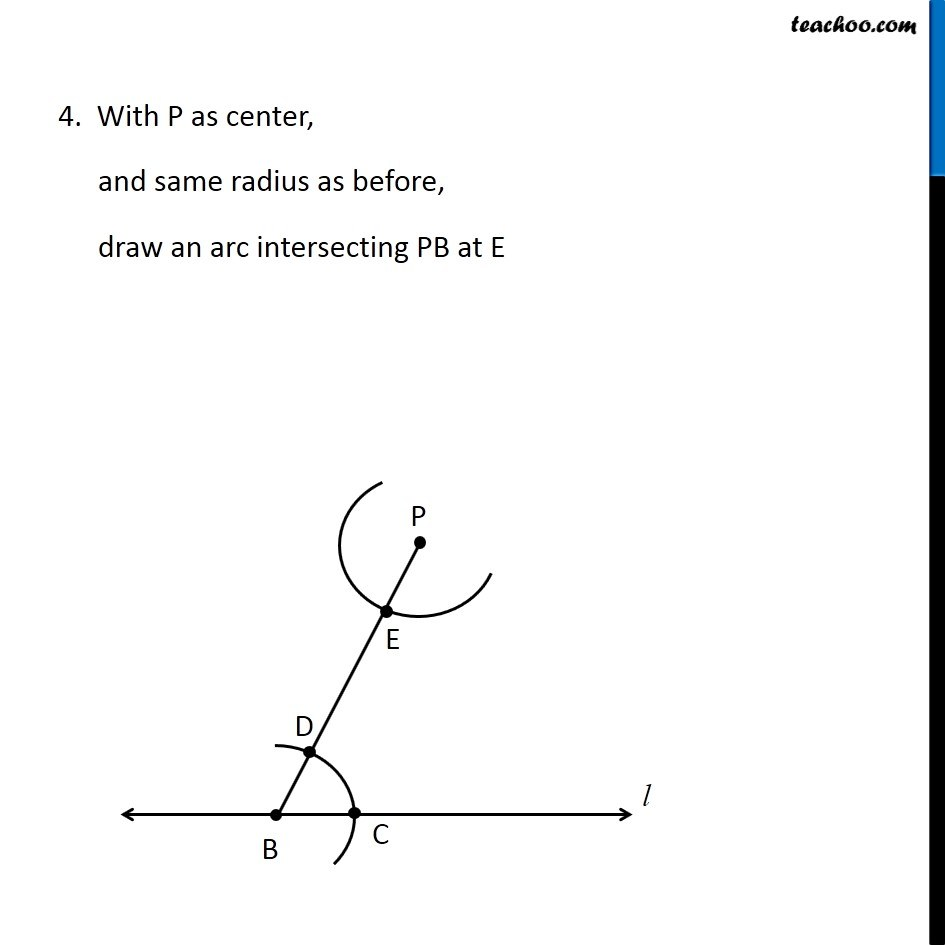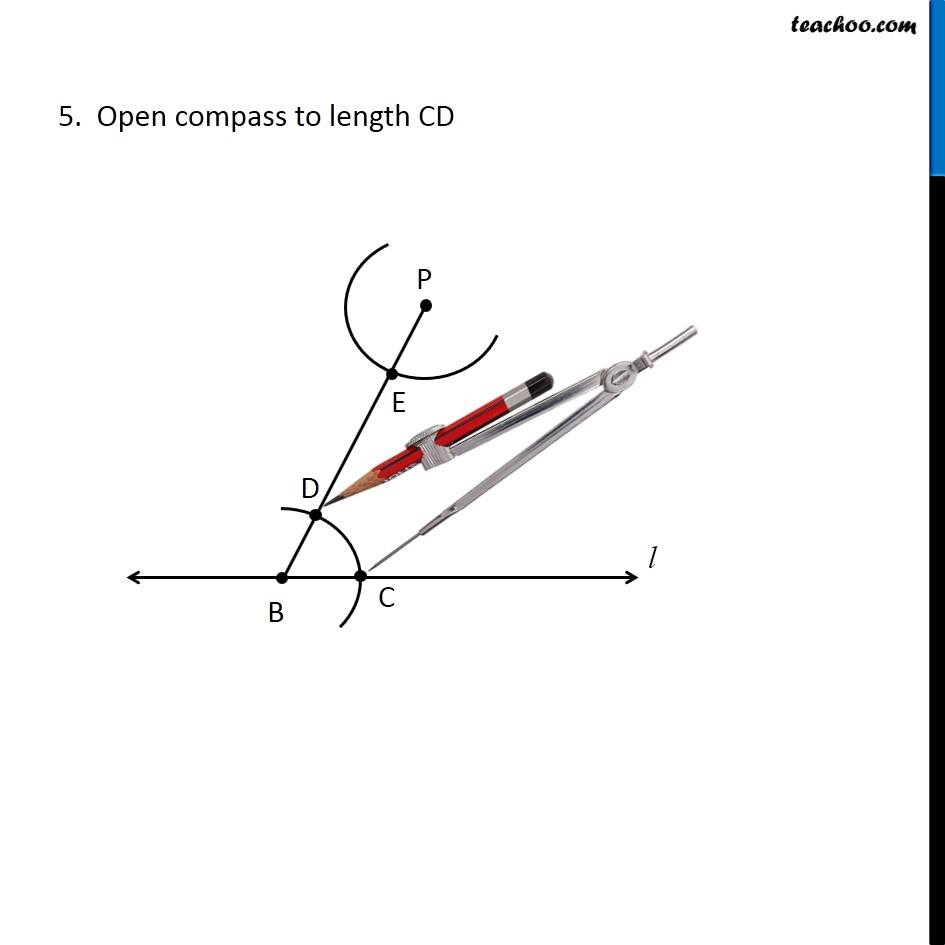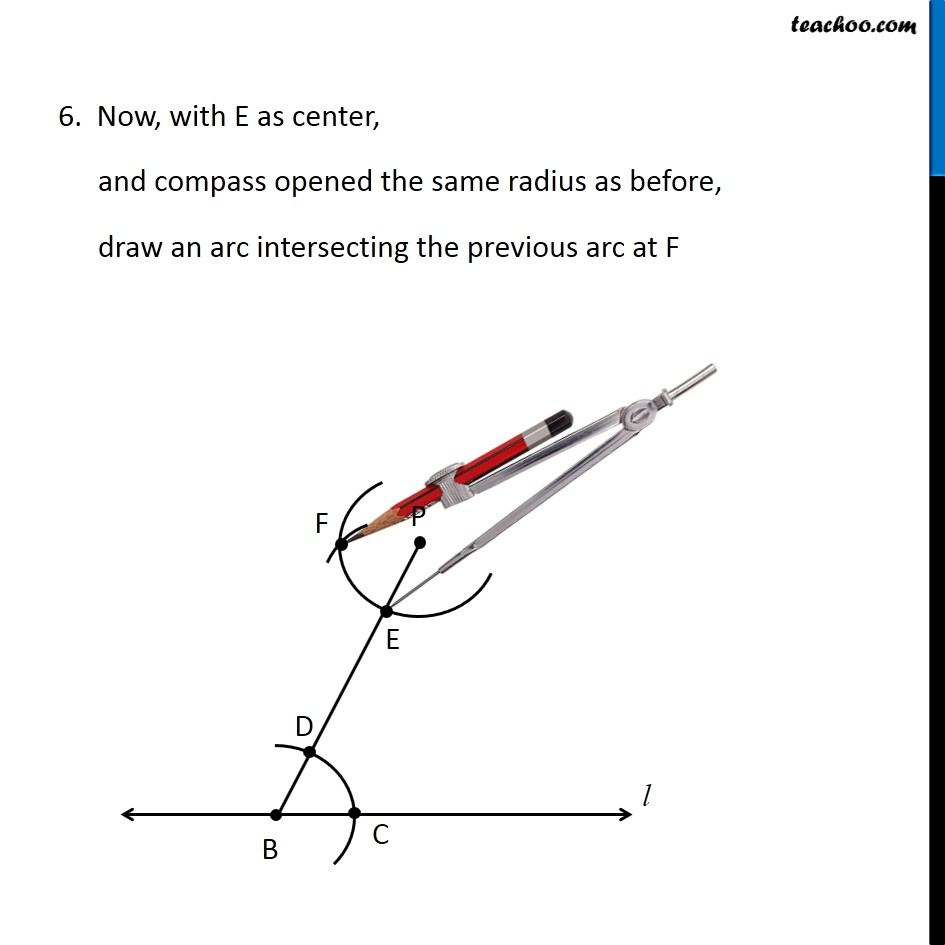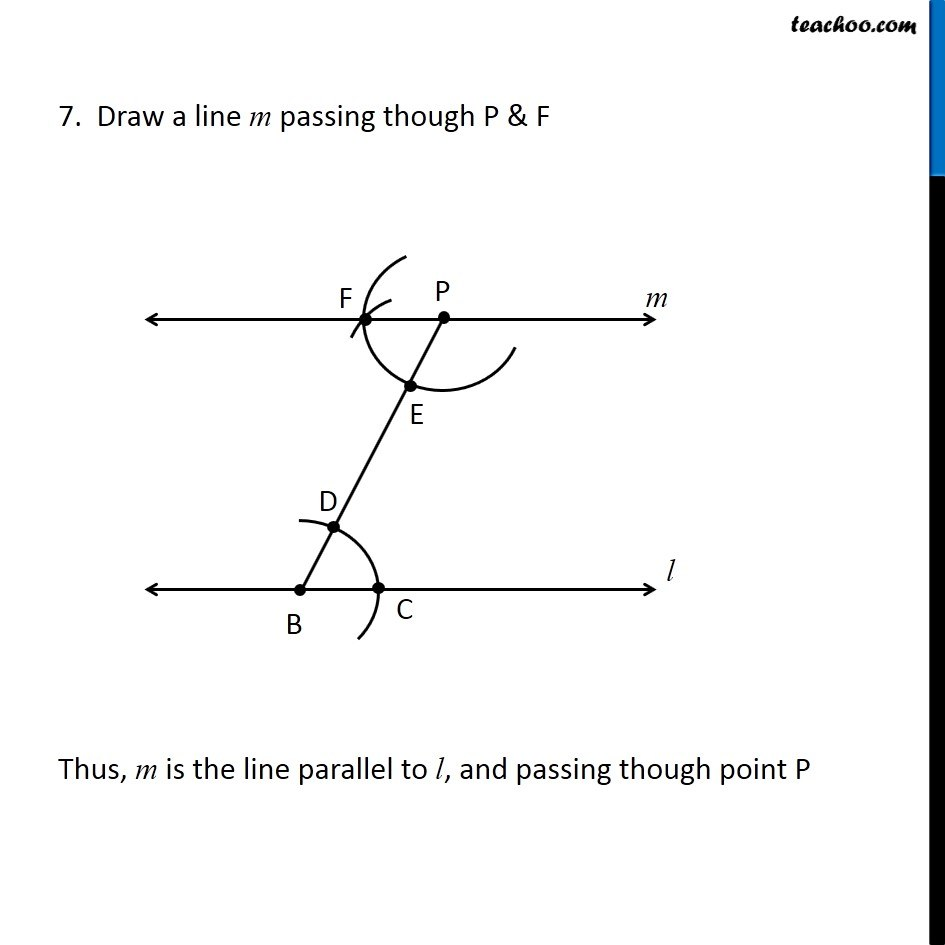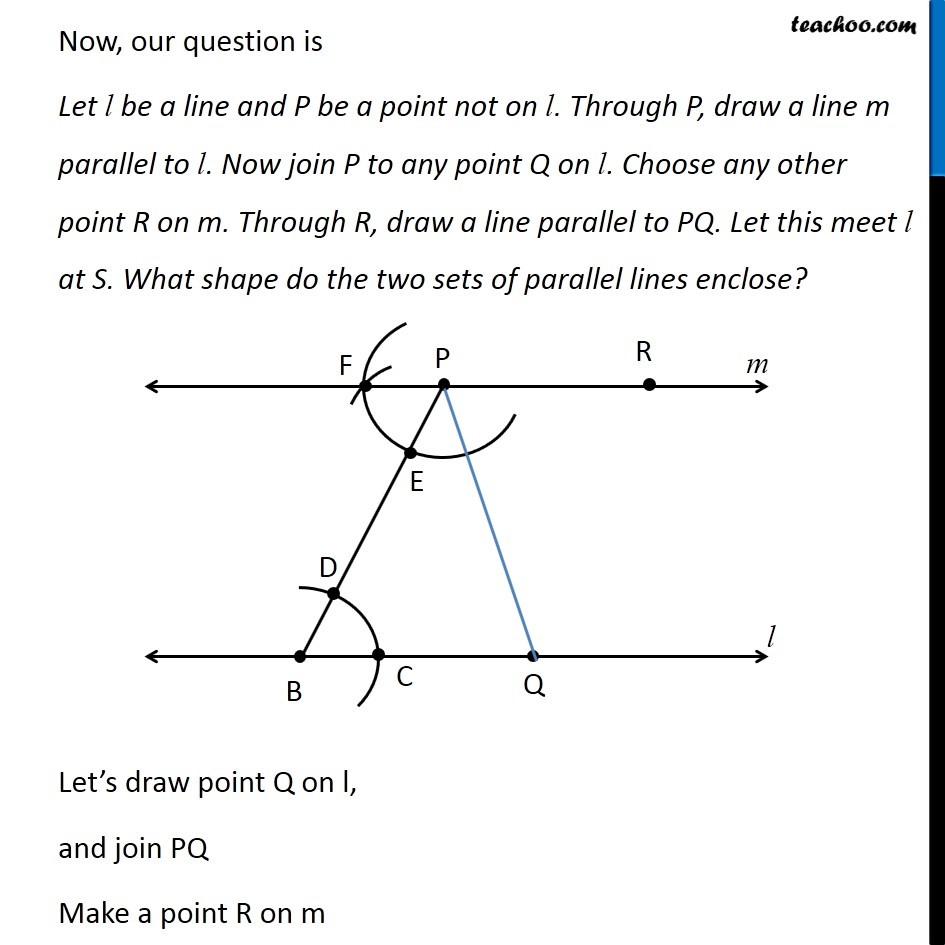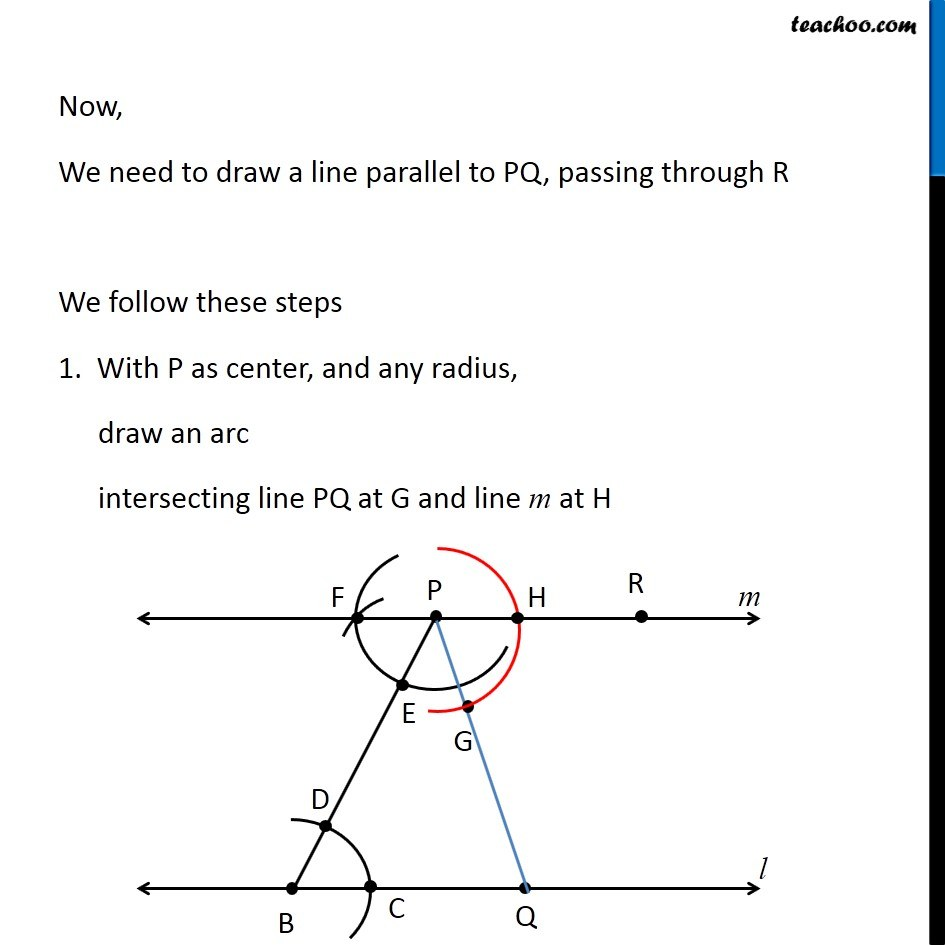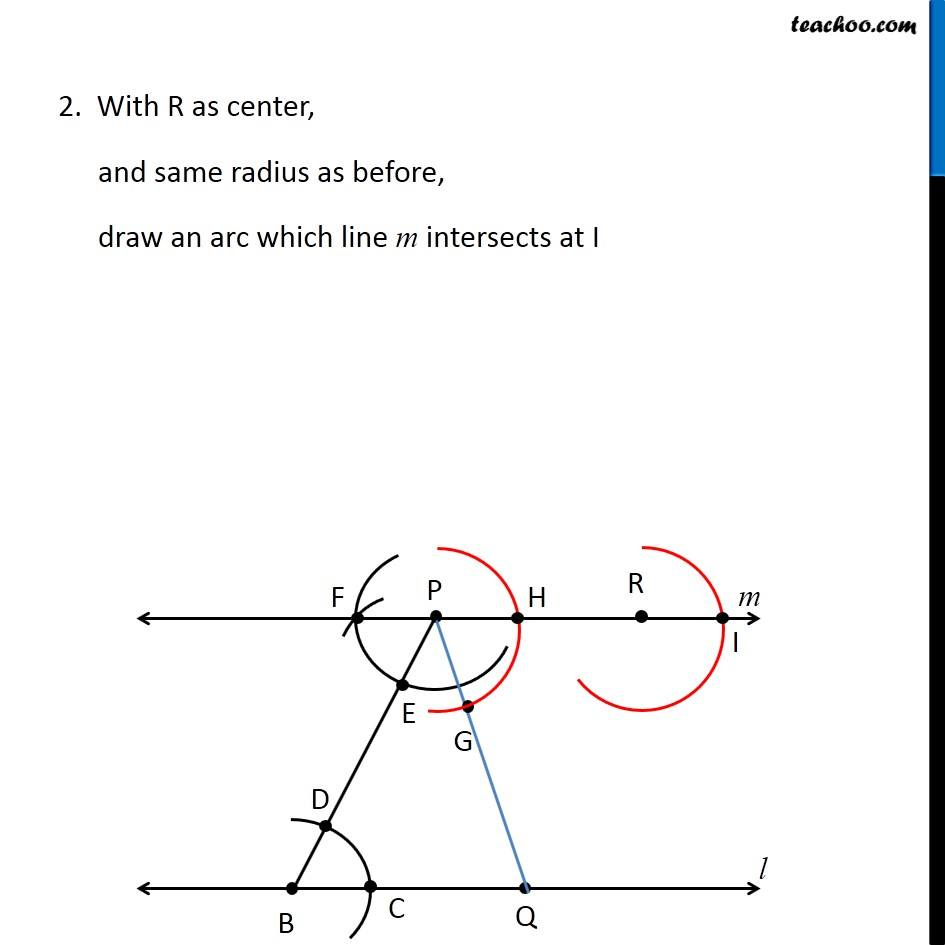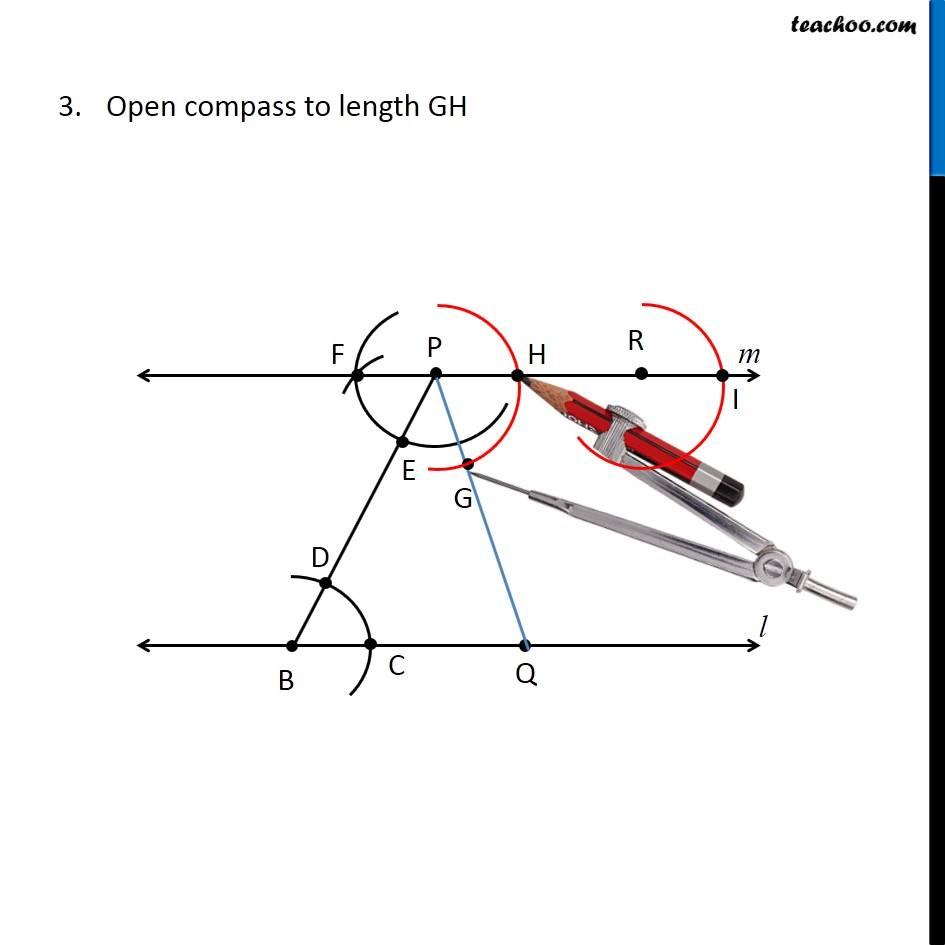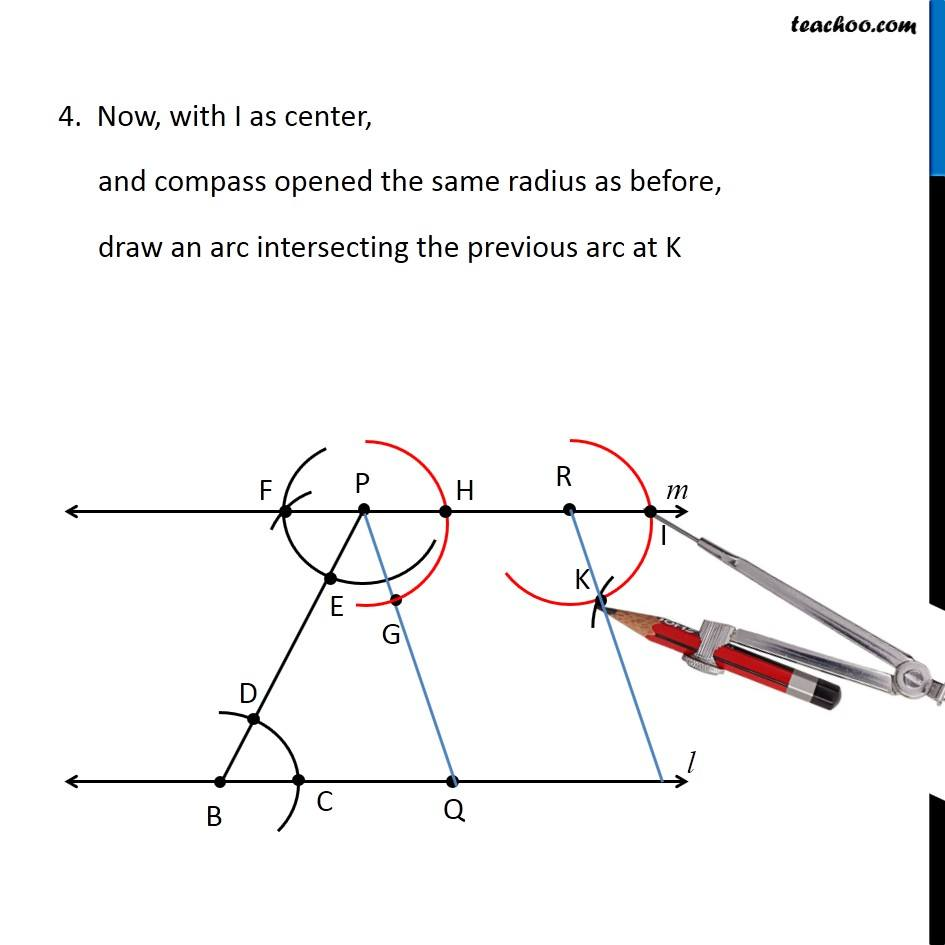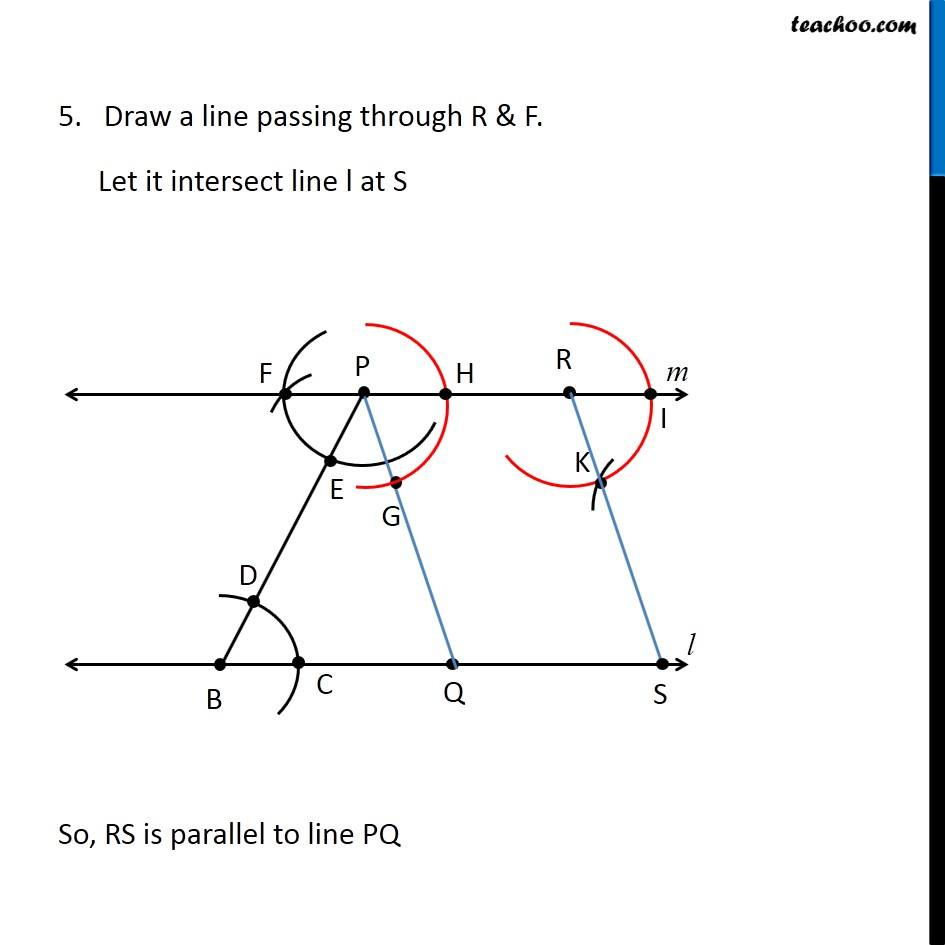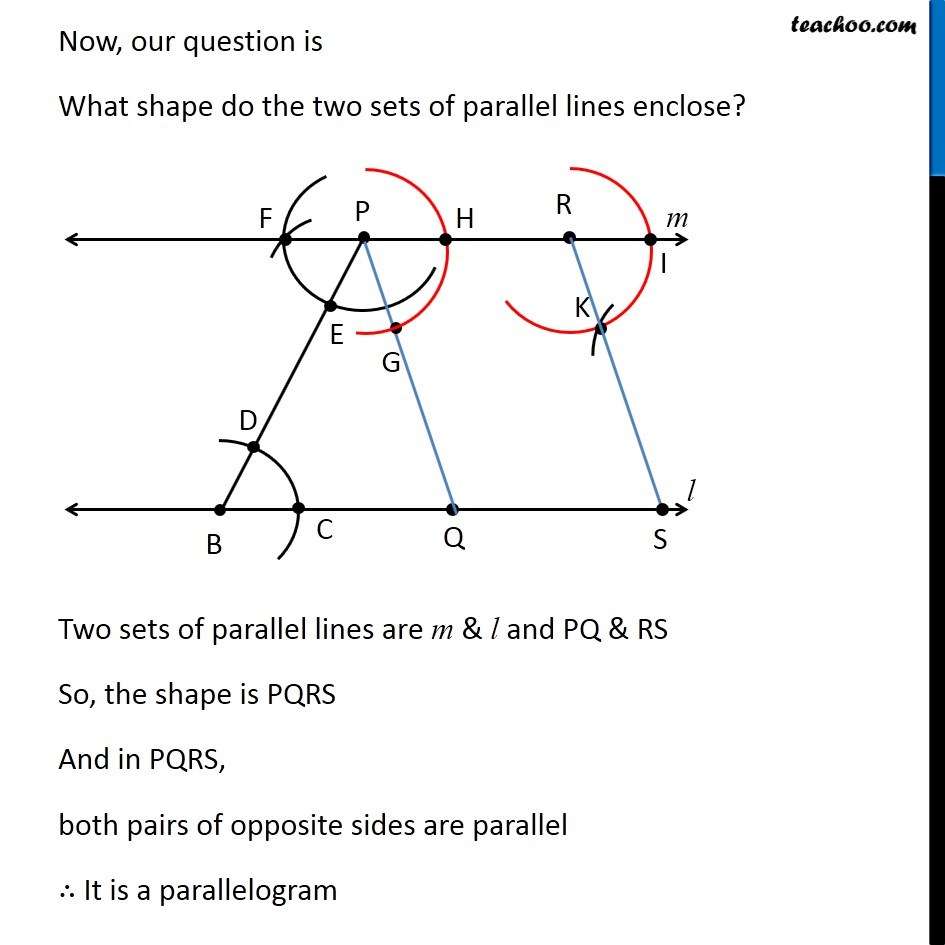Learn in your speed, with individual attention - Teachoo Maths 1-on-1 Class

### Transcript

Question 3 Let l be a line and P be a point not on l. Through P, draw a line m parallel to l. Now join P to any point Q on l. Choose any other point R on m. Through R, draw a line parallel to PQ. Let this meet l at S. What shape do the two sets of parallel lines enclose? Let’s first draw a line parallel to l, passing through point P 1. Given a line l with point P not on it 2. Mark point B on the line l Join PB 3. With B as center, and any radius, draw an arc intersecting l at C, and PB at D 4. With P as center, and same radius as before, draw an arc intersecting PB at E 5. Open compass to length CD 6. Now, with E as center, and compass opened the same radius as before, draw an arc intersecting the previous arc at F 7. Draw a line m passing though P & F Thus, m is the line parallel to l, and passing though point P Now, our question is Let l be a line and P be a point not on l. Through P, draw a line m parallel to l. Now join P to any point Q on l. Choose any other point R on m. Through R, draw a line parallel to PQ. Let this meet l at S. What shape do the two sets of parallel lines enclose? Let’s draw point Q on l, and join PQ Make a point R on m Let’s draw point Q on l, and join PQ Make a point R on m Let’s draw point Q on l, and join PQ Make a point R on m Open compass to length GH 4. Now, with I as center, and compass opened the same radius as before, draw an arc intersecting the previous arc at K 5. Draw a line passing through R & F. Let it intersect line l at S So, RS is parallel to line PQ Now, our question is What shape do the two sets of parallel lines enclose? Two sets of parallel lines are m & l and PQ & RS So, the shape is PQRS And in PQRS, both pairs of opposite sides are parallel ∴ It is a parallelogram